Courses

# Test: Integration By Substitution

## 5 Questions MCQ Test Mathematics (Maths) Class 12 | Test: Integration By Substitution

Description
This mock test of Test: Integration By Substitution for JEE helps you for every JEE entrance exam. This contains 5 Multiple Choice Questions for JEE Test: Integration By Substitution (mcq) to study with solutions a complete question bank. The solved questions answers in this Test: Integration By Substitution quiz give you a good mix of easy questions and tough questions. JEE students definitely take this Test: Integration By Substitution exercise for a better result in the exam. You can find other Test: Integration By Substitution extra questions, long questions & short questions for JEE on EduRev as well by searching above.
QUESTION: 1

###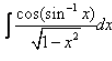Solution:

cos(sin-1x)/(1-x2)½……………….(1)
t = sin-1 x
dt = dx/(1-x2)½
Put the value of dt in eq(1)
= ∫cost dt
= sint + c
= sin(sin-1 x) + c
⇒ x + c

QUESTION: 2

### Integral of sin5x.cos2x is:

Solution:

So Looking at this integral, we have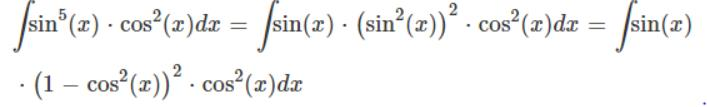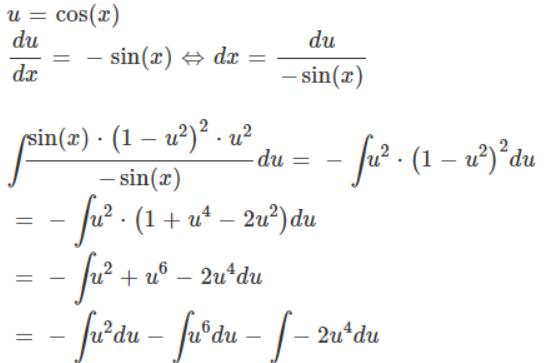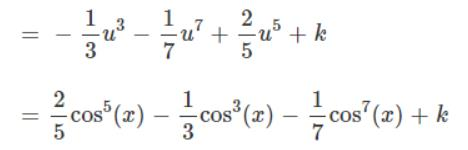QUESTION: 3

### Evaluate: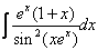Solution:

xex = t
(xex + ex) dx = dt
= ex(x + 1) dx = dt
= ∫dt/sin2t
= ∫cosec2t dt
= -cot(xex) + c

QUESTION: 4

Evaluate: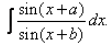Solution:

Create ((X+B) + (A-B)) in numerator and then apply Sin(a+b) formula then you will be able to solve it.

QUESTION: 5

Find the distance travelled by a car moving with acceleration given by a(t)=t2 + t, if it moves from t = 0 sec to t = 10 sec, if velocity of a car at t = 0sec is 40 km/hr.

Solution: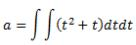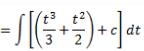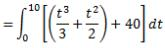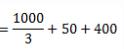= 783.3 km